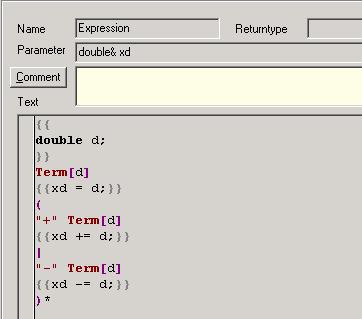Production: Expression
 Examples > Calculator > Production: Expression   The production: Expression has a parameter:Parameter: double& xd   This is the interface, where the variable, defined in the Calculator1 production, is put into the Expression production. Inside of the Expression production it has the new name xd. double again is the type of the variable. The "&" characterizes the variable as a reference, that means, that the value, which may be changed inside of the Expression production will accessible afterwards outside in the calling production (Calculator1). Without the "&" the variable would keep its value inside of the Calculator1 production, even if the value of xd inside of the Expression variable had changed.   Leaving out the actions, the rule simplifies to:   Term (   "+" Term | "-" Term )*    An expression is a term, to which an arbitrary number of other terms can be added or subtracted.   The instruction:   double d;   declares (as above) a new variable of the type double, which shall take the value of the term. The result of the first term will be assigned to the reference variable xd:   xd = d;   The values of the following terms will be added or subtracted:   xd += d; respectively xd -= d;   These expressions are a shorter notation for:   xd = xd + d; respectively xd = xd - d;